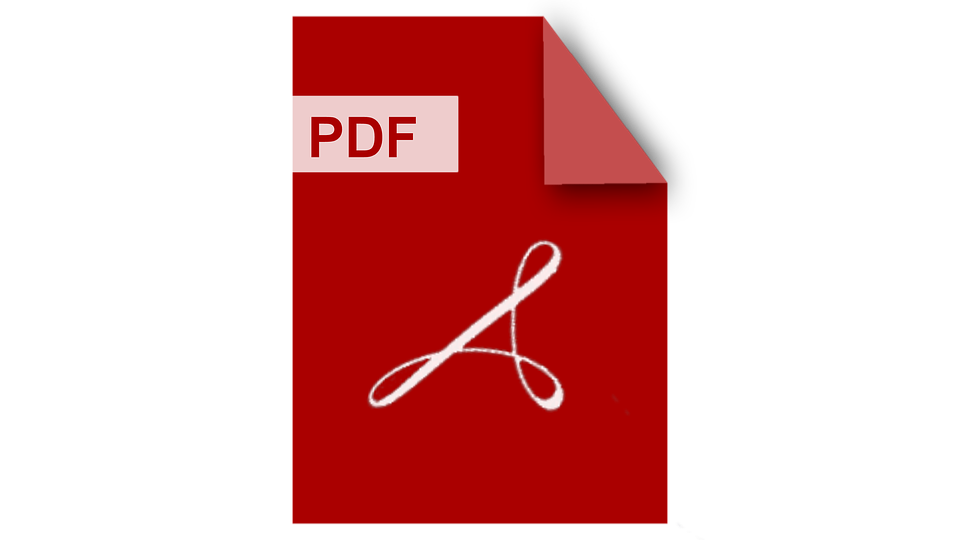WCSE 2021 SPRING ISBN: 978-981-18-1791-5
DOI: 10.18178/wcse.2021.02.005

## Optimal IIR Filter Based Equalizer Design via a Functional Inequality Constrained Optimization Approach

Yan Liang, Ling Wing-Kuen, Ya Li

Abstract— A finite impulse response (FIR) filter based equalizer requires a very high computational power and suffers from a long delay. On the other hand, an infinite impulse response (IIR) filter based equalizer can reduce a lot of computational cost for the implementation. This paper develops a joint model of a speaker and a transmission line via both the input audio signal and the input signal of the equalizer. The frequency response of the transmission system is modeled by the discrete time Fourier Transform of the input and output signal of the system. The modeled frequency response of the transmission system multiplies the frequency response of the equalizer should be approximately equal to 1 at every frequency point. To design these IIR filters, the total absolute errors between 1 and the modeled frequency response of the transmission system multiplies the frequency response of the corresponding IIR filters are minimized subject to the specifications on their maximum upper bounds and the stability condition of the equalizer. These problems are actually the functional inequality constrained optimization problems. By employing the constraint transcription method, these infinite constrained optimization problems can be converted to the finite constrained optimization problems and the solution is the coefficients of the IIR filter. The computer numerical simulation results show that the product of the frequency response of the developed joint model and that of the equalizer is small.

Index Terms— equalizer design, infinite impulse response filter design, functional inequality constrained optimization, infinite constrained optimization, constraint transcription

Yan Liang, Ling Wing-Kuen
Guangdong University of Technology, CHINA
Ya Li
Guangdong Polytechnic Normal University, CHINA[Download]

Cite: Yan Liang, Ling Wing-Kuen, Ya Li, "Optimal IIR Filter Based Equalizer Design via a Functional Inequality Constrained Optimization Approach, " Proceedings of 2021 the 11th International Workshop on Computer Science and Engineering (WCSE 2021), pp. 31-36, February 25-27, 2021.